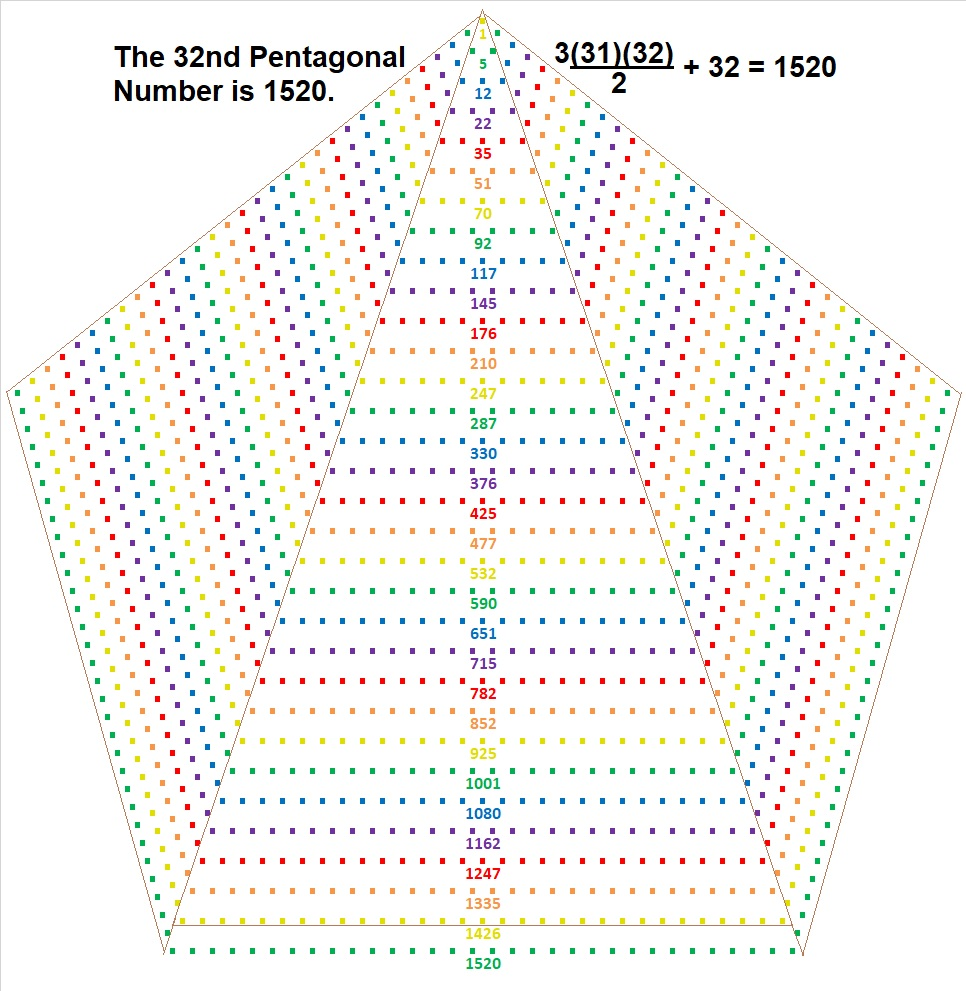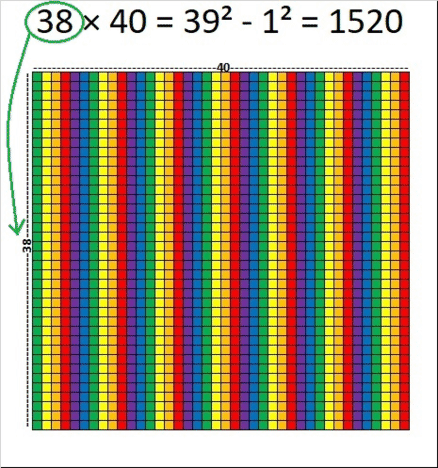# What Kind of Shape is 1520 in?

### Today’s Puzzle:

Sure, it’s a rectangle with whole-number sides in 10 different ways, but what kind of REGULAR polygonal shape can 1520 be made into? I will tell you that the measurement of each of its sides is 32.

And thus, it is the 32nd shape of its kind. By the way, I really like how all the 32nd figurate numbers relate to each other:We see in the chart that 1520 dots can be arranged into a pentagon. Just how do we do that? Here’s how:Do you see from the graphic that 1520 is 32 more than three times the 31st triangular number?

1520 is also related to triangular numbers in another way: Today I learned that all pentagonal numbers are 1/3 of a triangular number.  Indeed, 1520 is 1/3 of the 95th triangular number:
(1/3) of (95)(96)/2 = 1520.

Pretty cool, I think!

### Factors of 1520:

• 1520 is a composite number.
• Prime factorization: 1520 = 2 × 2 × 2 × 2 × 5 × 19, which can be written 1520 = 2⁴ × 5 × 19
• 1520 has at least one exponent greater than 1 in its prime factorization so √1520 can be simplified. Taking the factor pair from the factor pair table below with the largest square number factor, we get √1520 = (√16)(√95) = 4√95. The exponents in the prime factorization are 4, 1, and 1. Adding one to each exponent and multiplying we get (4 + 1)(1 + 1)(1 + 1) = 5 × 2 × 2 = 20. Therefore 1520 has exactly 20 factors.
• The factors of 1520 are outlined with their factor pair partners in the graphic below.### More about the Number 1520:

If you look at the whole thread, you will see how a few people explained this important concept using arrays. Here is my attempt to explain the difference of two squares using 1520 and arrays:

As I mentioned before, 1520 has 10 rectangles with whole-number sides. The one with the smallest perimeter is 38 × 40, and it is the easiest to use to demonstrate how 1520 is the difference of two squares:make science GIFs like this at MakeaGif

I made that gif be as slow as I could without duplicating any of the frames, but it still goes pretty fast.

1520 is, in fact, the difference of two squares in six different ways:
39² – 1² = 1520,
48² – 28² = 1520,
81² – 71² = 1520,
99² – 91² = 1520,
192² – 188² = 1520, and
381² – 379² = 1520.

1520 is also the hypotenuse of a Pythagorean triple:
912-1216-1520, which is (3-4-5) times 304.

From OEIS.org we learn that 1520 and 1521 are a Ruth-Aaron pair because they are consecutive numbers and the sum of their factors are equal to each other:
1520 = 2⁴·5·19 and 1521 = 3²·13², while
2+2+2+2+5+19 = 32=3+3+13+13.